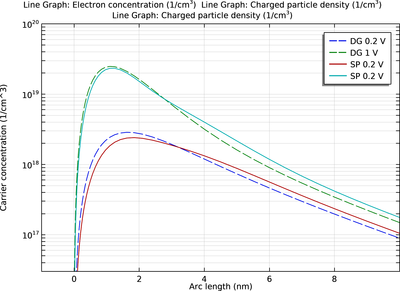# Application Gallery

## Density-Gradient and Schrödinger–Poisson Results for a Silicon Inversion Layer

Application ID: 73381

This tutorial demonstrates the use of the density-gradient formulation to include the effect of quantum confinement in the device physics simulation of a silicon inversion layer. This formulation requires only a moderate increase of computational resources as compared to the conventional drift-diffusion equation. Thus it allows much speedier engineering investigation than other more sophisticated quantum mechanical methods. The result of the density-gradient theory is compared with the solution of the Schrödinger-Poisson equation. The computed electron density profiles from both theories show the expected behavior of quantum confinement and both agree well with the published figures in the reference paper.This model example illustrates applications of this type that would nominally be built using the following products: